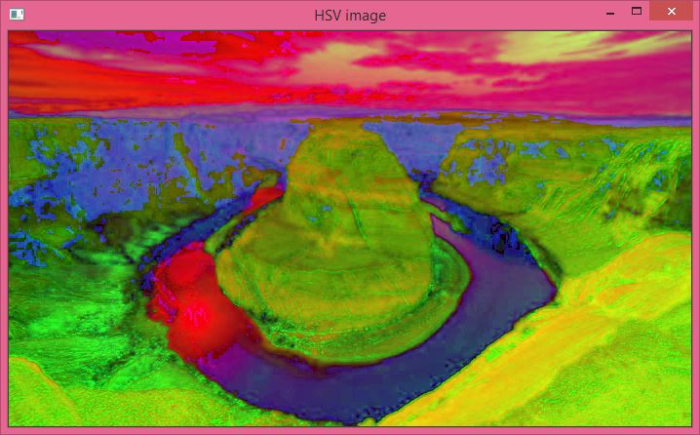# How to convert an RGB image to HSV image using OpenCV Python?

An RGB (colored) image has three channels, Red, Blue and Green. A colored image in OpenCV has a shape in [H, W, C] format, where H, W, and C are image height, width and number of channels. All three channels have a value range between 0 and 255.

The HSV image also has three channels, the Hue, Saturation and Value channels. In OpenCV, the values of the Hue channel range from 0 to 179, whereas the Saturation and Value channels range from 0 to 255.

In OpenCV, to convert an RGB image to HSV image, we use the cv2.cvtColor() function. This function is used to convert an image from one color space to another.

This function takes two arguments− first the input image and second the color conversion method. See the syntax given below −

cv2.cvtColor(bgr_img, cv2.COLOR_BGR2HSV)


## Steps

To convert an RGB image to HSV image, follow the steps given below −

Import the required library. In all the following Python examples, the required Python library is OpenCV. Make sure you have already installed it.

import cv2


Read the input RGB image using cv2.imread(). The RGB image read using this method is in BGR format. Optionally assign the read BGR image to bgr_img.

bgr_img = cv2.imread('water.jpg')


Now convert this BGR image to HSV image as below using cv2.cvtColor() function. Optionally assign the converted HSV image to hsv_img.

hsv_img = cv2.cvtColor(bgr_img, cv2.COLOR_BGR2HSV)


Display the above converted HSV image.

cv2.imshow('HSV image', hsv_img)
cv2.waitKey(0)
cv2.destroyAllWindows()


## Input Image

We will use this image as the input file in the following example.## Example

This Python program converts an RGB image to HSV image.

import cv2
# read the input RGB image as BGR format

# Convert the BGR image to HSV Image
hsv_img = cv2.cvtColor(bgr_img, cv2.COLOR_BGR2HSV)
cv2.imwrite('hsv_image.jpg', hsv_img)

# Display the HSV image
cv2.imshow('HSV image', hsv_img)
cv2.waitKey(0)
cv2.destroyAllWindows()


## Output

When you run the above program, it will produce the following outputNotice the difference between the original RGB image and the HSV image.